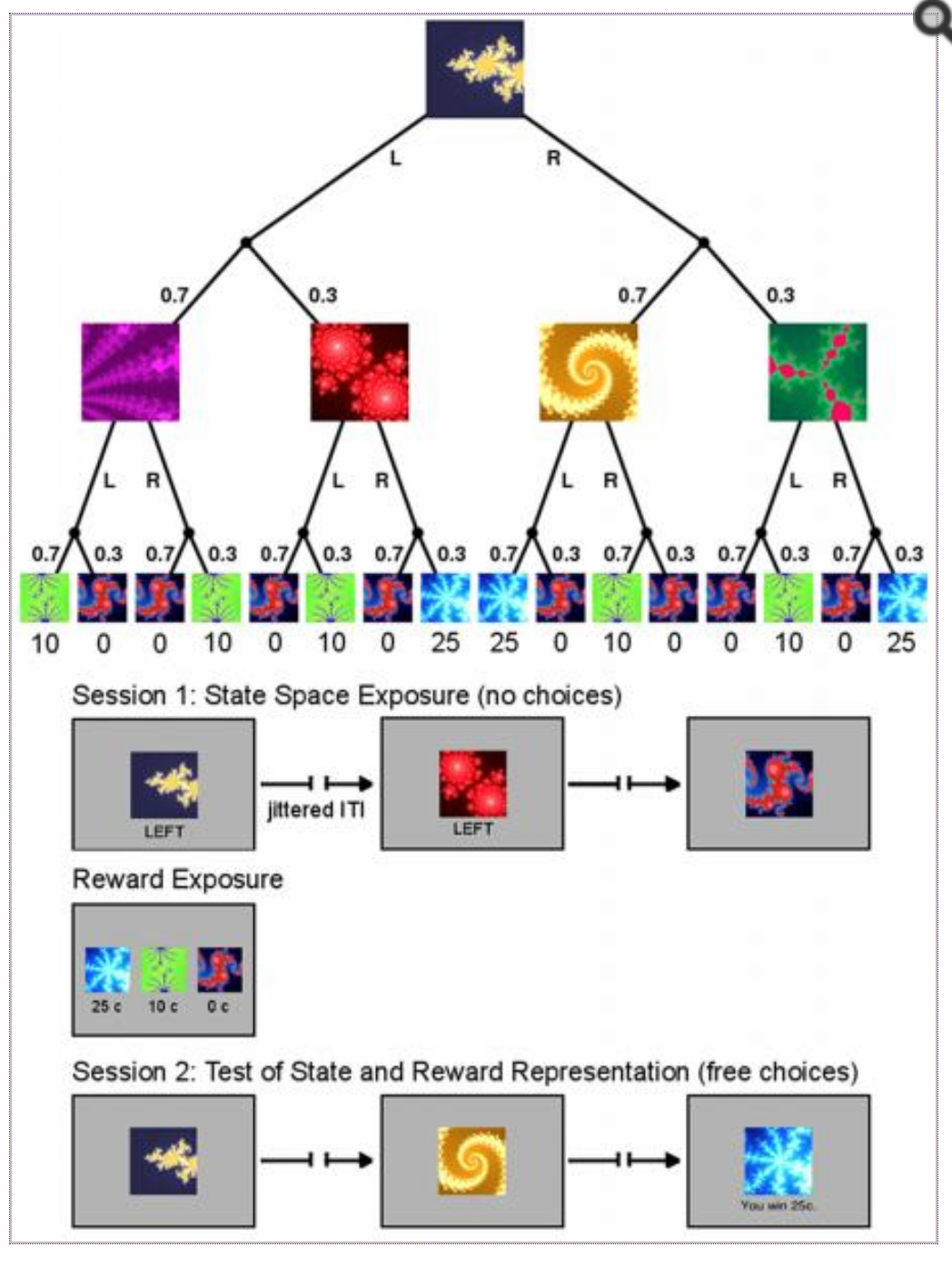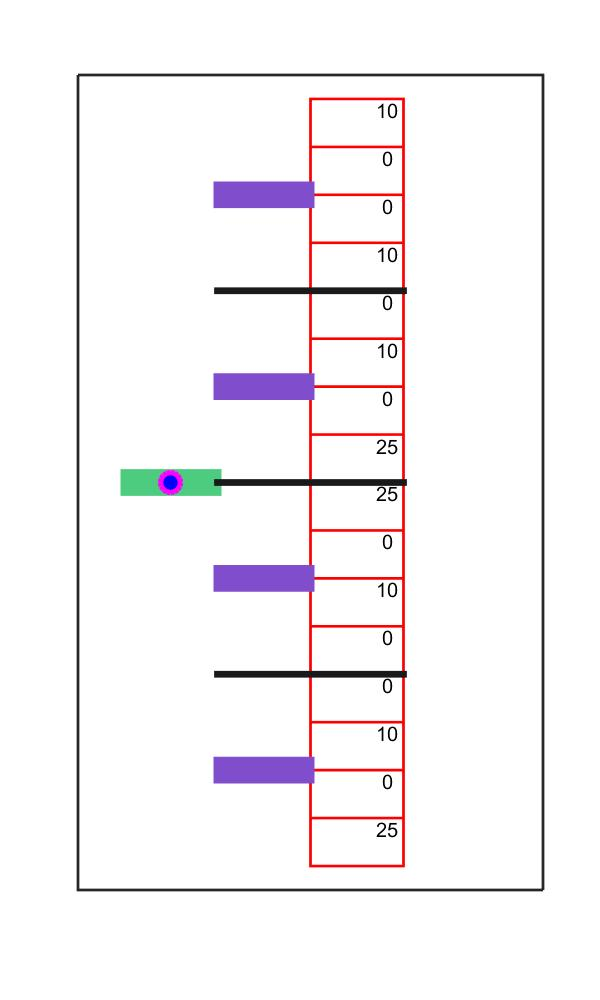# Model-based and Model-free RL solving a sequential two-choice Markov decision task

In this example I replicated task and model described in Glasher et al. 2010 (available here: https://www.ncbi.nlm.nih.gov/pmc/articles/PMC2895323/ ). The task is essentially a two armed bandit with probabilistic outcomes (distribution of probabilities: 0.7-0.3), played on two levels, so that the agent has to perform 2 choices in sequence (left or right), to reach a reward, virtually following the branches of a binary decision three. The rewards are static and they are represented by values of 0, 10 and 25.

If the behavior of the agent is controlled only by the model-free component (e.g. SARSA, see: example 1 or example 2), the agent will be able to discriminate correctly which action is associated with the highest expected values, at the time of the second choice. However, the model-free control alone would consider both actions at the first level as equally valuable, as if the overall rewards that can be reached after either initial choice were the same.5Thus, to solve the task it is necessary to rely on a hybrid control system that integrates the classic model-free with a decision making system capable of generating a correct map of state-action associations, that includes the different probabilities to navigate either task. This component is usually termed model-based, as it generates a model of the world on which choices are then based.You can download the whole code here (zip archive), where I have also added a graphical live representation (see below) of the choices performed by the agent, to allow easy track of the behaviour. Convergence towards optimal behavior across a short number of trial is not always found.

RL_two_choice_markovInsert math as
$${}$$